# How to insert an equation with integral

Word
This tip displays how to add an equation with integral, for example, Gauss's law, also known as Gauss's flux theorem.

How to add an equation in your document, see Working with Microsoft Equation.

To add an integral form of the Gauss's law, do the following:

## In the Professional format:

2.   On the Equation tab, in the Structures group, click the Integral button: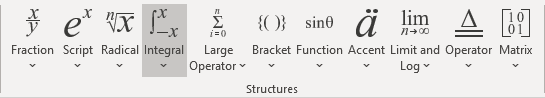In the Integral list, choose Contour Integral: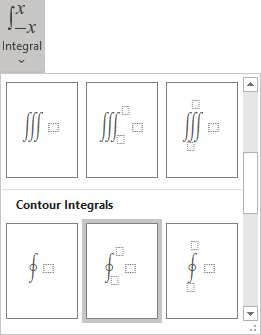3.   In the integral template:

• In the lower box, enter S.
• In the upper box, right-click and choose Hide Upper limit in the popup menu: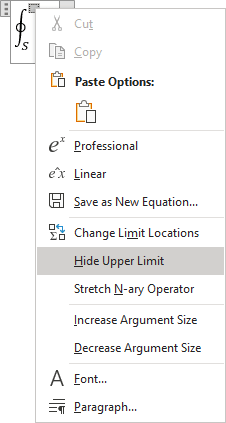• In the right base box, enter E.

4.   On the Equation tab, in the Symbols group, in the list of symbols choose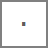.

5.   Then enter dA=.

6.   On the Equation tab, in the Structures group, click the Fraction button. In the Fraction list choose Stacked Fraction: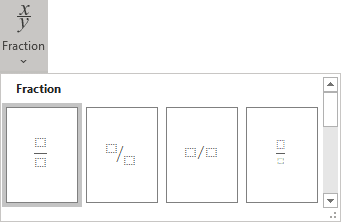7.   Enter Q at the top of your fraction.

8.   In the bottom of your fraction, do the following:

• On the Equation tab, in the Structures group, click the Script button.
• In the Script list, choose Subscript: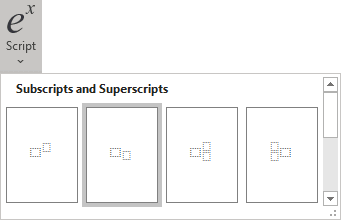• On the Equation tab, in the Symbols group, in the list of symbols choose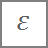,
• In the lower box of the subscript, enter 0.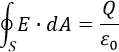## In the Linear format:

2.   Do one of the following:

• On the Equation tab, in the Symbols group, click the More button: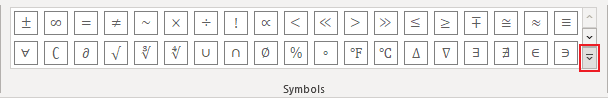In the top of the list of symbols, choose Operators: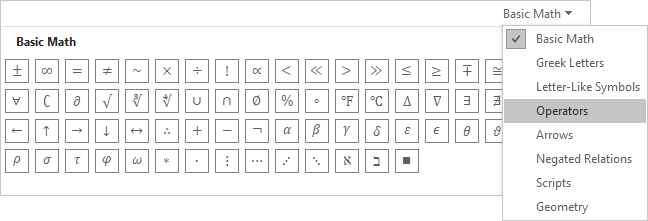In the Operators list, choose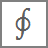.
• Simply enter \oint.

3.   Enter _S. Then you enter a space key; this linear formula transformed into a professional format.

4.   In the base box of integral, enter E.

5.   On the Equation tab, in the Symbols group, choose(or simply enter \bullet).

6.   Then enter dA=Q/, choose(or simply enter \varepsilon) and then _0: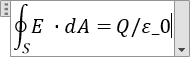Then you enter a space key, your linear formula transformed into the professional format.